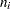The BOXPLOT Procedure
 Input Data Sets

### DATA= Data Set

You can read analysis variable measurements from a data set specified with the DATA= option in the PROC BOXPLOT statement. Each analysis variable specified in the PLOT statement must be a SAS variable in the data set. This variable provides measurements that are organized into groups indexed by the group variable. The group variable, specified in the PLOT statement, must also be a SAS variable in the DATA= data set. Each observation in a DATA= data set must contain a value for each analysis variable and a value for the group variable. If theth group containsmeasurements, there should beconsecutive observations for which the value of the group variable is the index of theth group. For example, if each group contains 20 items and there are 30 groups, the DATA= data set should contain 600 observations. Other variables that can be read from a DATA= data set include the following:

• block variables

• symbol variable

• BY variables

• ID variables

### BOX= Data Set

You can read group summary statistics and outlier information from a BOX= data set specified in the PROC BOXPLOT statement. This enables you to reuse OUTBOX= data sets that have been created in previous runs of the BOXPLOT procedure to reproduce schematic box plots.

A BOX= data set must contain the following variables:

• the group variable

• _VAR_, containing the analysis variable name

• _TYPE_, identifying features of box-and-whiskers plots

• _VALUE_, containing values of those features

Each observation in a BOX= data set records the value of a single feature of one group’s box-and-whiskers plot, such as its mean. Consequently, a BOX= data set contains multiple observations per group. These must appear consecutively in the BOX= data set.

The _TYPE_ variable identifies the feature whose value is recorded in a given observation. The following table lists valid _TYPE_ variable values.

Table 24.8 Valid _TYPE_ Values in a BOX= Data Set

_TYPE_

Description

N

group size

MIN

group minimum value

Q1

group first quartile

MEDIAN

group median

MEAN

group mean

Q3

group third quartile

MAX

group maximum value

STDDEV

group standard deviation

LOW

low outlier value

HIGH

high outlier value

LOWHISKR

low whisker value, if different from MIN

HIWHISKR

high whisker value, if different from MAX

FARLOW

low far outlier value

FARHIGH

high far outlier value

The features identified by _TYPE_ values N, MIN, Q1, MEDIAN, MEAN, Q3, and MAX are required for each group.

Other variables that can be read from a BOX= data set include the following:

• the variable _ID_, containing labels for outliers

• the variable _HTML_, containing URLs to be associated with features on box plots

• block variables

• symbol variable

• BY variables

• ID variables

When you specify the keyword SCHEMATICID or SCHEMATICIDFAR with the BOXSTYLE= option, values of _ID_ are used as outlier labels. If _ID_ does not exist in the BOX= data set, the values of the first variable listed in the ID statement are used.

### HISTORY= Data Set

You can read group summary statistics from a HISTORY= data set specified in the PROC BOXPLOT statement. This enables you to reuse OUTHISTORY= data sets that have been created in previous runs of the BOXPLOT procedure or to read output data sets created with SAS summarization procedures, such as PROC UNIVARIATE.

Note that a HISTORY= data set does not contain outlier information. Therefore, in general you cannot reproduce a schematic box plot from summary statistics saved in an OUTHISTORY= data set. To save and reproduce schematic box plots, use OUTBOX= and BOX= data sets.

A HISTORY= data set must contain the following:

• the group variable

• a group minimum variable for each analysis variable

• a group first-quartile variable for each analysis variable

• a group median variable for each analysis variable

• a group mean variable for each analysis variable

• a group third-quartile variable for each analysis variable

• a group maximum variable for each analysis variable

• a group standard deviation variable for each analysis variable

• a group size variable for each analysis variable

The names of the group summary statistics variables must be the analysis variable name concatenated with the following special suffix characters.

Group Summary Statistic

Suffix Character

group minimum

L

group first quartile

1

group median

M

group mean

X

group third quartile

3

group maximum

H

group standard deviation

S

group size

N

For example, consider the following statements:

```proc boxplot history=Summary;
plot (Weight Yieldstrength) * Batch;
run;
```

The data set Summary must include the variables Batch, WeightL, Weight1, WeightM, WeightX, Weight3, WeightH, WeightS, WeightN, YieldstrengthL, Yieldstrength1, YieldstrengthM, YieldstrengthX, Yieldstrength3, YieldstrengthH, YieldstrengthS, and YieldstrengthN.

Note that if you specify an analysis variable whose name contains the maximum of 32 characters, the summary variable names must be formed from the first 16 characters and the last 15 characters of the analysis variable name, suffixed with the appropriate character.

These other variables can be read from a HISTORY= data set:

• block variables

• symbol variable

• BY variables

• ID variablesPrevious Page | Next Page | Top of Page